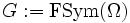# Finitary symmetric group on countable set is subgroup-universal for finite groups

This article gives the statement, and possibly proof, of an embeddability theorem: a result that states that any group of a certain kind can be embedded in a group of a more restricted kind.
View a complete list of embeddability theorems

## Statement

Let$\Omega$ be a countably infinite set and$G := \operatorname{FSym}(\Omega)$ be the finitary symmetric group on$\Omega$. Then,$G$ is subgroup-universal for finite groups. In other words, if$H$ is any finite group,$H$ is isomorphic to a subgroup of$G$.

## Facts used

1. Cayley's theorem: This states that every group embeds as a subgroup of the symmetric group on its underlying set.

## Proof

The proof follows from fact (1), and the observation that the symmetric group on any finite set is isomorphic to some subgroup of$G$ -- namely, the subgroup comprising the permutations on a finite subset of$\Omega$ of the same cardinality.